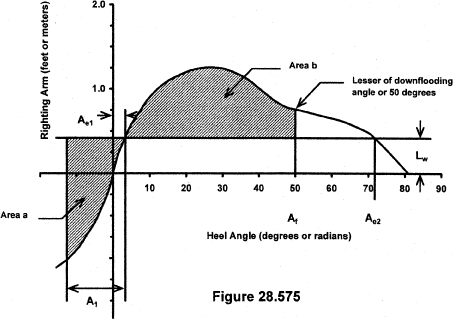# Title 46

## SECTION 28.575

### 28.575 Severe wind and roll.

§ 28.575 Severe wind and roll.

(a) Each vessel must meet paragraphs (f) and (g) of this section when subjected to the gust wind heeling arm and the angle of roll to windward as specified in this section.

(b) The gust wind heeling arm, Lw in figure 28.575 of this chapter, must be calculated by the following formula:

KEn(Vn 2AnZn)/W where: K = 0.00216 when consistent English units are used or 1.113 when consistent metric units are used. En = series summation notation where n varies from 1 to the number of elements in the series; Vn = S[0.124LN(0.3048hn) + 0.772], in feet per second S[0.127LN(hn) + 0.772], in meters per second and is the wind speed for profile element “n” on a vessel; S = 64 (19.5, if metric units are used) for a vessel that operates on protected waters; or 85.3 (26, if metric units are used) for a vessel that operates on waters other than protected waters; LN = natural logarithm; hn = the vertical distance from the centroid of area An to the waterline for profile element n, in feet (meters); An = projected lateral area for profile element n, in square feet (square meters); Zn = the vertical distance between the centroid of An and a point at the center of the underwater lateral area or a point at approximately one-half of the draft, for profile element n, in feet; and W = displacement of the loaded vessel, in pounds (Newtons).

(c) The angle of roll to windward, A1, is measured from the equilibrium angle, Ael, and is calculated by the following formula:

A1 = 109kXY[Square root of (rs)], in degrees, where: s,X,Y = factors from table 28.575; r = 0.73 + 0.6 Zg/d; Zg = distance between the center of gravity and the waterline (+ above, − below), in feet (meters); k = 1.0 for round bilged vessels with no bilge keels or bar keels; 0.7 for vessels with sharp bilges, or the value from table 28.575 for vessels with a bar keel, bilge keels, or both; B = molded breadth of the vessel, in feet (meters); d = mean molded draft of the vessel, in feet (meters); Cb = block coefficient; Ak = aggregate area of bilge keels, the area of the lateral projection of a bar keel, or the sum of these areas, in square feet (square meters); L = length, in feet (meters); T = 1.108 BC/square root of GM, in seconds; 2.0 BC/square root of GM, if metric units are used; GM = metacentric height corrected for free surface effects, as explained in § 28.540, in feet (meters); C = 0.373 + 0.023(B/d)−0.000131L or 0.373 + 0.023(B/D)−0.00043L, if metric units are used.

(d) The angle of equilibrium, Ael in figure 28.575, is calculated by determining the lowest angle at which the gust wind heeling arm, Lw, is equal to the righting arm.

(e) The area “b” in figure 28.575 must be measured to the least of the following:

(1) The angle of downflooding, (Af);

(2) The angle of the second intercept, Ae2 in figure 28.575, of the wind heeling arm curve, Lw in figure 28.575, and the righting arm curve; or

(3) A heel angle of 50° (0.87 radians).

(f) The angle of equilibrium, Ael in figure 28.575, must not exceed 14° (0.24 radians).

(g) Area “b” in figure 28.575 must not be less than area “a” in figure 28.575.

Tables 28.575 - Roll Factors

B/d X
2.4 1.0
2.5 0.98
2.6 0.96
2.7 0.95
2.8 0.93
2.9 0.91
3.0 0.90
3.1 0.88
3.2 0.86
3.3 0.84
3.4 0.82
3.5 0.80

Note. Intermediate values must be obtained by interpolation.

Cb Y
0.45 0.75
0.50 0.82
0.55 0.89
0.60 0.95
0.93 0.97
0.70 1.0

Note. Intermediate values must be obtained by interpolation.

100Ak/(LB) k
0 1.0
1.0 0.98
1.5 0.95
2.0 0.88
2.5 0.79
3.0 0.74
3.5 0.72
4.0 0.70

Note. Intermediate values must be obtained by interpolation.

T S
6 0.100
7 0.098
8 0.093
12 0.065
14 0.053
16 0.044
18 0.038
20 0.035

Note: Intermediate values must be obtained by interpolation.[56 FR 40393, Aug. 14, 1991, CGD 88-079; 56 FR 47679, Sept. 20, 1991, CGD 88-079, as amended by CGD 95-072, 60 FR 50461, Sept. 29, 1995; USCG-2004-18884, 69 FR 58344, Sept. 30, 2004; USCG-2008-0906, 73 FR 56509, Sept. 29, 2008]